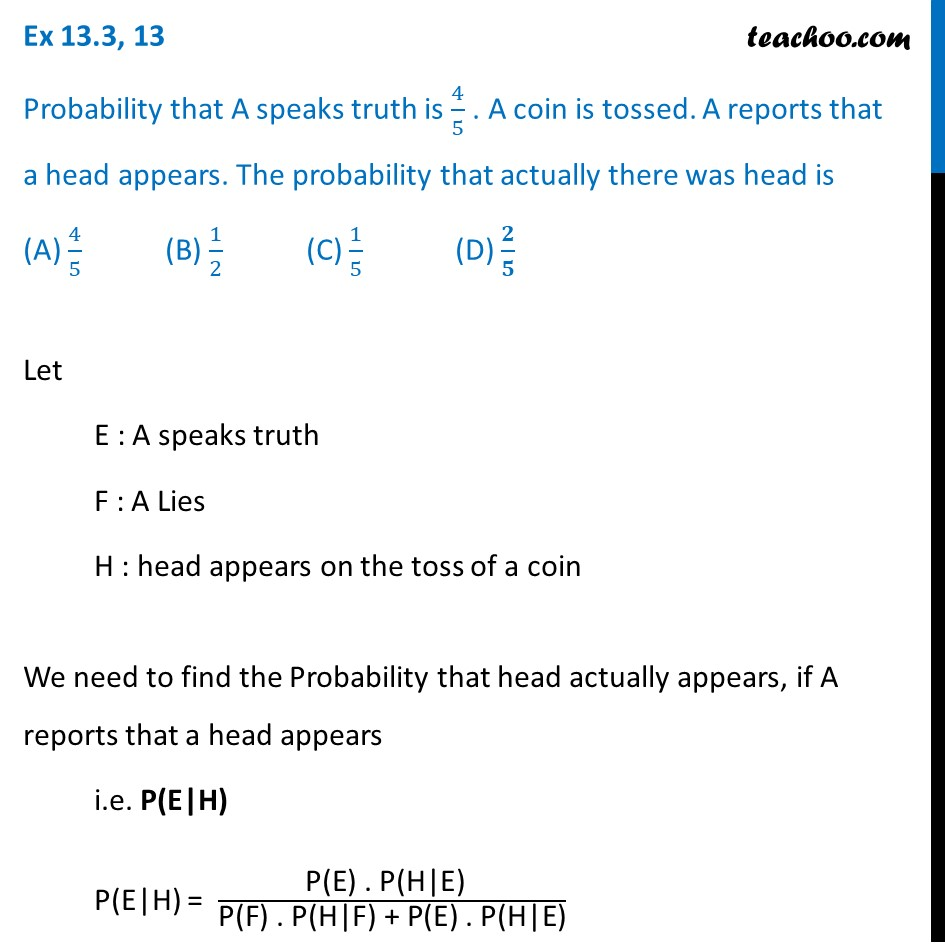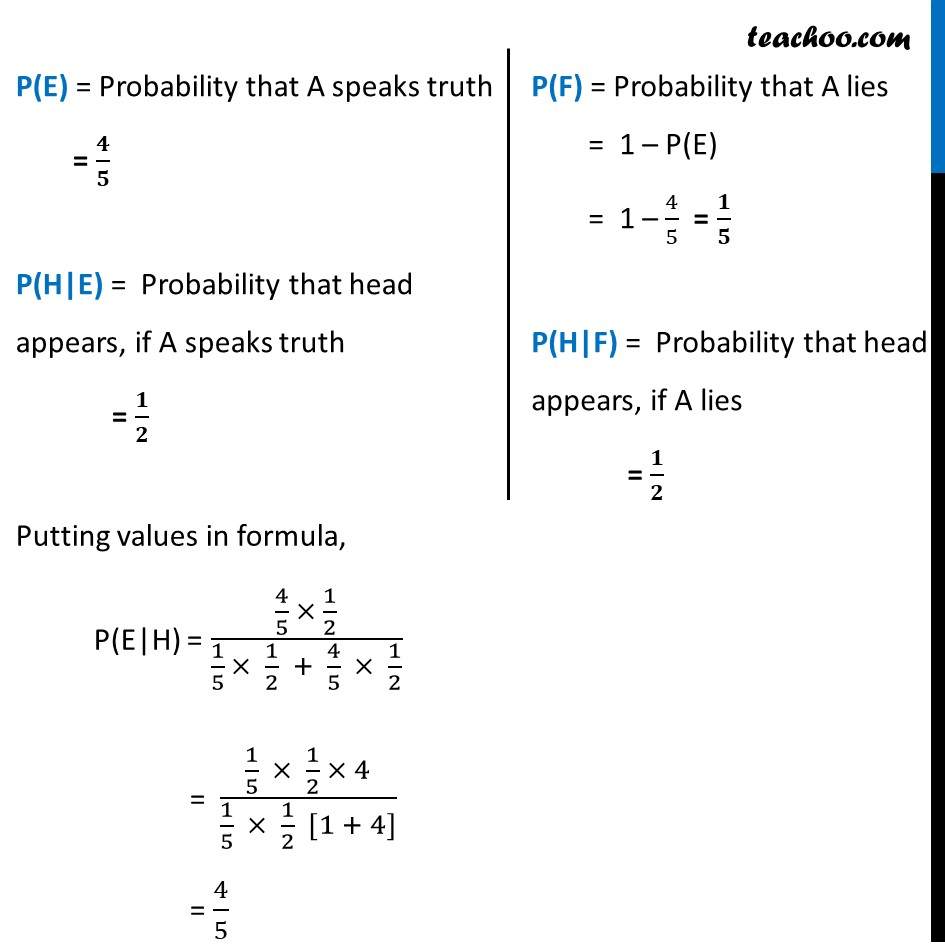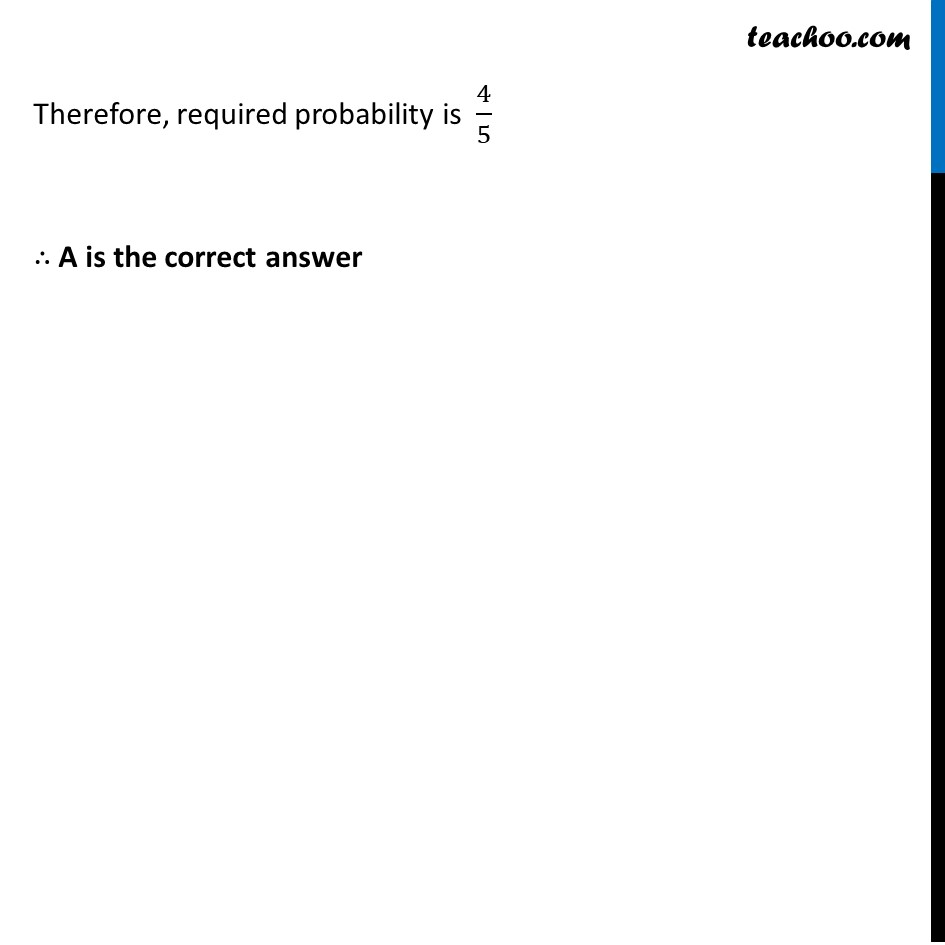Ex 13.3

Chapter 13 Class 12 Probability
Serial order wiseLearn in your speed, with individual attention - Teachoo Maths 1-on-1 Class

### Transcript

Ex 13.3, 13 Probability that A speaks truth is 4/5 . A coin is tossed. A reports that a head appears. The probability that actually there was head is (A) 4/5 (B) 1/2 (C) 1/5 (D) 𝟐/𝟓Let E : A speaks truth F : A Lies H : head appears on the toss of a coin We need to find the Probability that head actually appears, if A reports that a head appears i.e. P(E|H) P(E|H) = "P(E) . P(H|E) " /"P(F) . P(H|F) + P(E) . P(H|E)" P(E) = Probability that A speaks truth = 𝟒/𝟓 P(H|E) = Probability that head appears, if A speaks truth = 𝟏/𝟐 P(F) = Probability that A lies = 1 – P(E) = 1 – 4/5 = 𝟏/𝟓 P(H|F) = Probability that head appears, if A lies = 𝟏/𝟐 Putting values in formula, P(E|H) = (4/5 × 1/2)/(1/5 × 1/2 + 4/5 × 1/2) = (1/5 × 1/2 × 4)/(1/5 × 1/2 [1 + 4]) = 4/5 Therefore, required probability is 4/5 ∴ A is the correct answer